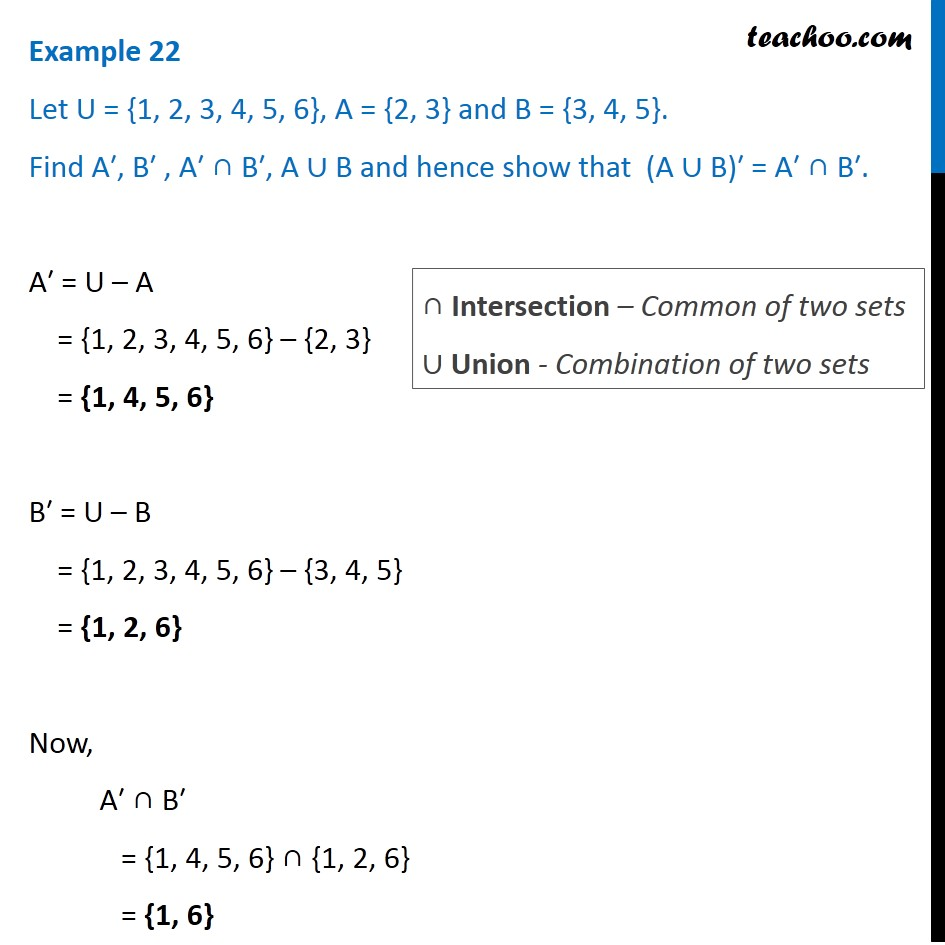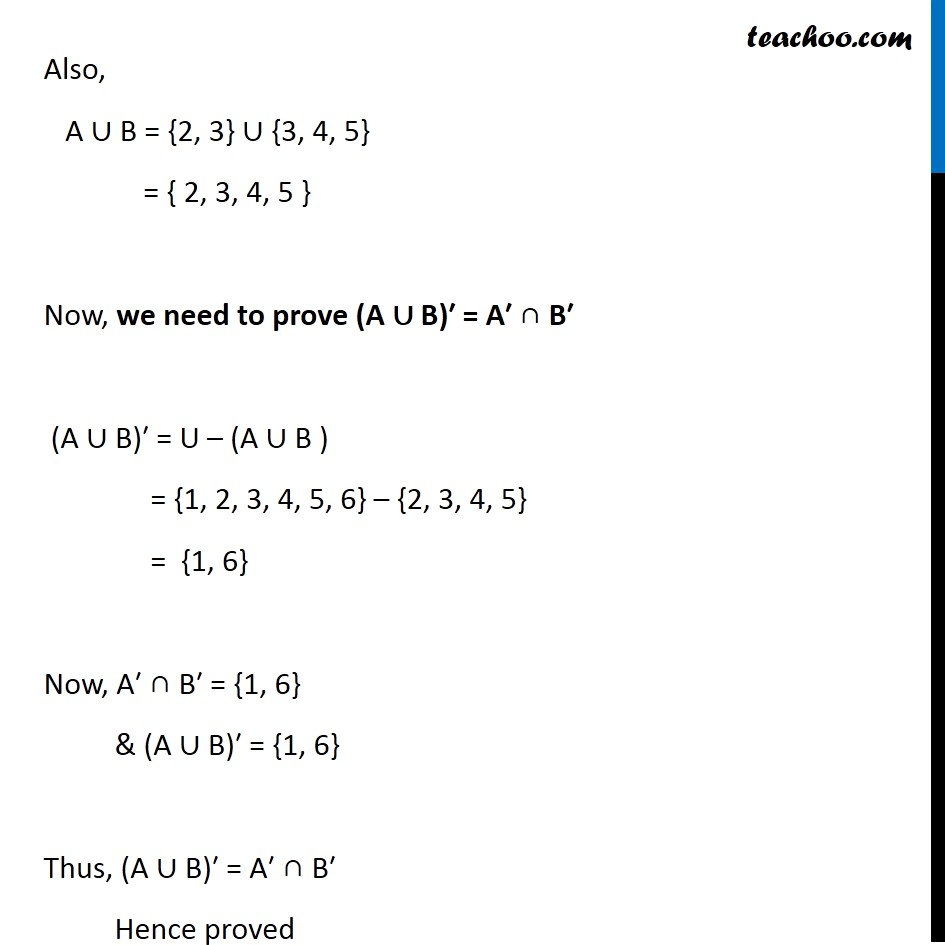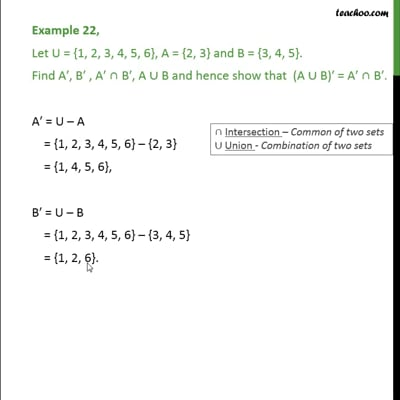Complement of set

Chapter 1 Class 11 Sets
Concept wiseThis video is only available for Teachoo black users

Introducing your new favourite teacher - Teachoo Black, at only ₹83 per month

### Transcript

Example 22 Let U = {1, 2, 3, 4, 5, 6}, A = {2, 3} and B = {3, 4, 5}. Find A′, B′ , A′ ∩ B′, A ∪ B and hence show that (A ∪ B)′ = A′ ∩ B′. A′ = U – A = {1, 2, 3, 4, 5, 6} – {2, 3} = {1, 4, 5, 6} B′ = U – B = {1, 2, 3, 4, 5, 6} – {3, 4, 5} = {1, 2, 6} Now, A′ ∩ B′ = {1, 4, 5, 6} ∩ {1, 2, 6} = {1, 6} ∩ Intersection – Common of two sets ∪ Union - Combination of two sets Also, A ∪ B = {2, 3} ∪ {3, 4, 5} = { 2, 3, 4, 5 } Now, we need to prove (A ∪ B)′ = A′ ∩ B′ (A ∪ B)′ = U – (A ∪ B ) = {1, 2, 3, 4, 5, 6} – {2, 3, 4, 5} = {1, 6} Now, A′ ∩ B′ = {1, 6} & (A ∪ B)′ = {1, 6} Thus, (A ∪ B)′ = A′ ∩ B′ Hence proved